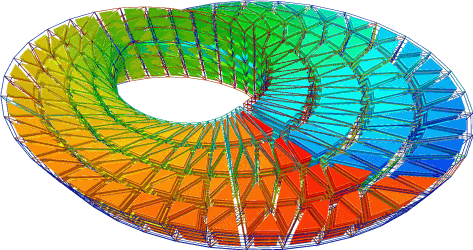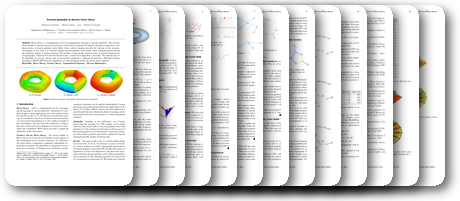## Towards optimality in discrete Morse theoryThomas Lewiner, Hélio Lopes, Geovan Tavares

Experimental Mathematics 12(3): pp. 271-285 (december 2003)

## Abstract:

Morse theory is a fundamental tool for investigating the topology of smooth manifolds. This tool has been extended to discrete structures by Forman, which allows combinatorial analysis and direct computation. This theory relies on discrete gradient vector fields, whose critical elements describe the topology of the structure. The purpose of this work is to construct optimal discrete gradient vector fields, where optimality means having the minimum number of critical elements. The problem is equivalently stated in terms of maximal hyperforests of hypergraphs. Deduced from this theoretical result, a construction algorithm is provided. The optimal parts are proved, and the part of exponential complexity is replaced by heuristics. Although reaching optimality is MAX-SNP hard, the experiments on odd topological models are almost optimal.

PDF paper (2 MB)## BibTeX:

@article{morse_optim_expmath,
author = {Thomas Lewiner and Hélio Lopes and Geovan Tavares},
title = {Towards optimality in discrete Morse theory},
year = {2003},
month = {december},
journal = {Experimental Mathematics},
volume = {12},
number = {3},
pages = {271--285},
publisher = {A.K.Peters},
doi = {10.1080/10586458.2003.10504498},
url = {\url{http://thomas.lewiner.org/pdfs/morse_optim_expmath.pdf}}
}

Last modifications on July 3rd, 2013